# XPLOR XPLOR minimizes the hybrid energy function Ehybrid

• Slides: 16X-PLOR XPLOR minimizes the hybrid energy function Ehybrid = Echem = wexp. Eexp where Echem could be a molecular dynamics force field (CHARMM, AMBER, OPLS/AMBER) a modified geometric force field Engh/Huber, PROLSQ, PARALLHDG and Eexp would be derived from Xray data or NMR data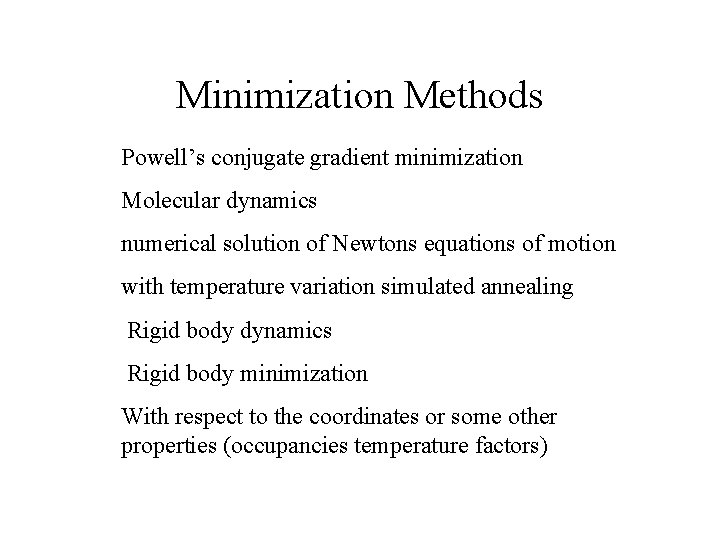Minimization Methods Powell’s conjugate gradient minimization Molecular dynamics numerical solution of Newtons equations of motion with temperature variation simulated annealing Rigid body dynamics Rigid body minimization With respect to the coordinates or some other properties (occupancies temperature factors)Step 1: define topology (chemical (primary) structure) topology @TOPPAR: topallhdg. pro end segment name= “ “ chain @TOPPAR: tophpep 19. pro sequence Ala end end write structure output=diala. psf end stopCalculate Energies To minimize we need PSF file (Structure) structure @diala. psf end energy parameters parameter @TOPPAR: parallhdg. pro end starting coordinates XPLOR PDB format coor @diala. pdb end mini powell nstep=50 end write coor output=dialamin. pdb end stopEnergy FunctionMolecular Dynamics Molecular dynamics is the numerical solution of Newton’s equations of motion Fi = mi ai = mi d 2 xi/dt 2 = d/dxi ETOTAL second order differential equation masses mi are defined in topology file ETOTAL is the XPLOR energy function force field in parameter file and experimental terms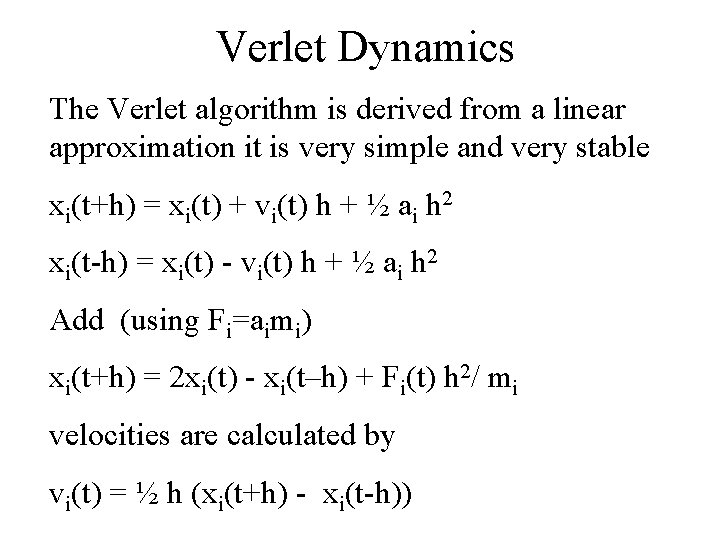Verlet Dynamics The Verlet algorithm is derived from a linear approximation it is very simple and very stable xi(t+h) = xi(t) + vi(t) h + ½ ai h 2 xi(t-h) = xi(t) - vi(t) h + ½ ai h 2 Add (using Fi=aimi) xi(t+h) = 2 xi(t) - xi(t–h) + Fi(t) h 2/ mi velocities are calculated by vi(t) = ½ h (xi(t+h) - xi(t-h))Langevin Dynamics XPLOR can calculate Langevin dynamics mi d 2 xi/dt 2(t) = -gradxi E + [ fi(t) ]- mibi dxi/dt(t) in addition to Newton equation friction terms fi is a random force on atom i mibi dxi/dt(t) is a velocity dependent friction term with friction constant bi and is used as temperature control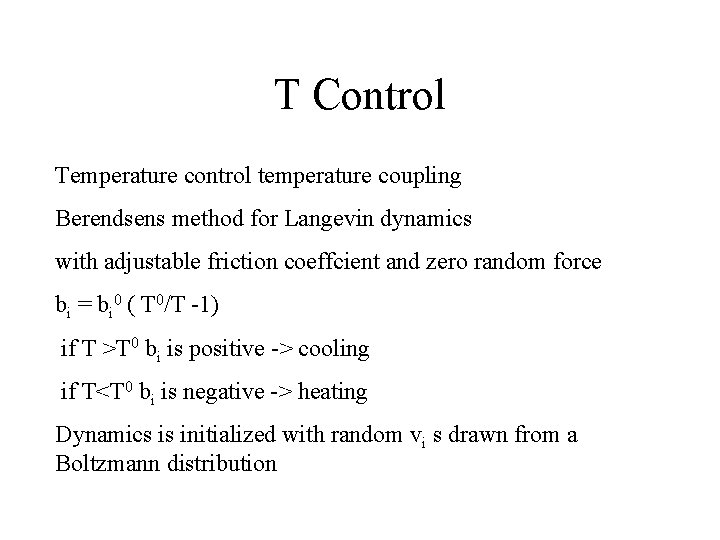T Control Temperature control temperature coupling Berendsens method for Langevin dynamics with adjustable friction coeffcient and zero random force bi = bi 0 ( T 0/T -1) if T >T 0 bi is positive -> cooling if T<T 0 bi is negative -> heating Dynamics is initialized with random vi s drawn from a Boltzmann distributionSlow Cooling A slow cooling script … evaluate (\$bath = 1000) vector do (vx = \$bath) (all) vector do (vy = \$bath) (all) vector do (vz = \$bath) (all) while (\$bath > 50) loop cool evaluate (\$bath = \$bath - \$tempstep) dynamics verlet nstep=1000 time=0. 005 iasvel=current tcoup=true tbath=\$bath nprint=\$nstep iprfrq=\$ntrfrq end loop cool …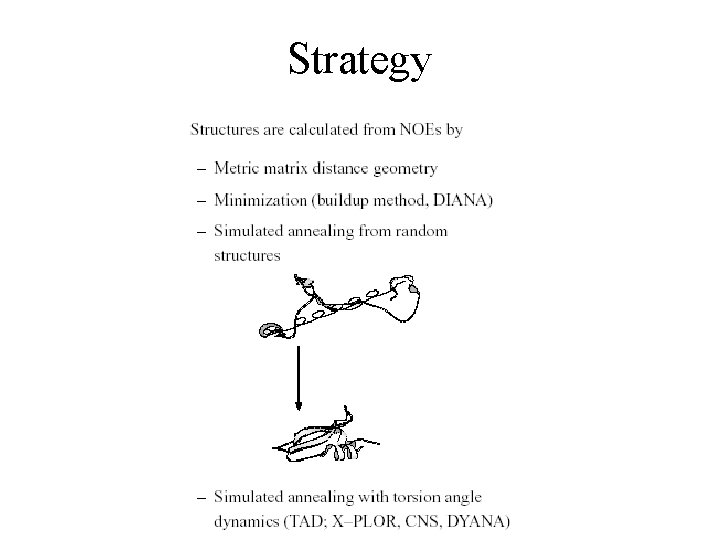Strategy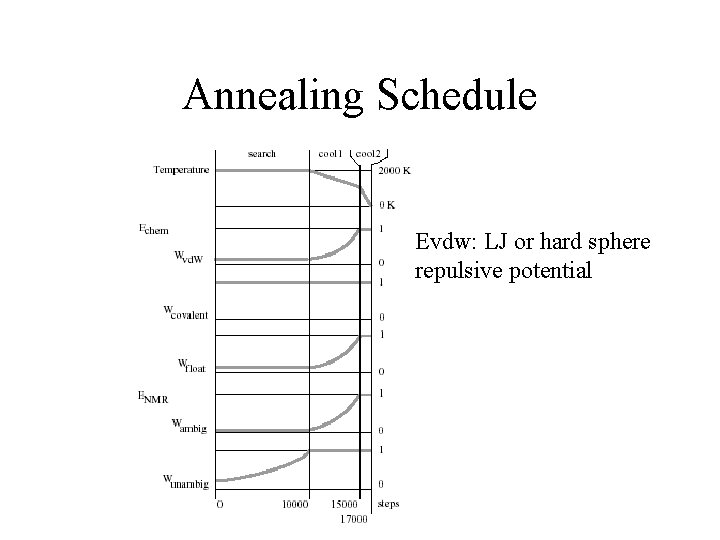Annealing Schedule Evdw: LJ or hard sphere repulsive potentialE(NOE) asymptote k(r-rupper) constant force harmonic region (r-rupper)2 flat bottom [rlower –rupper]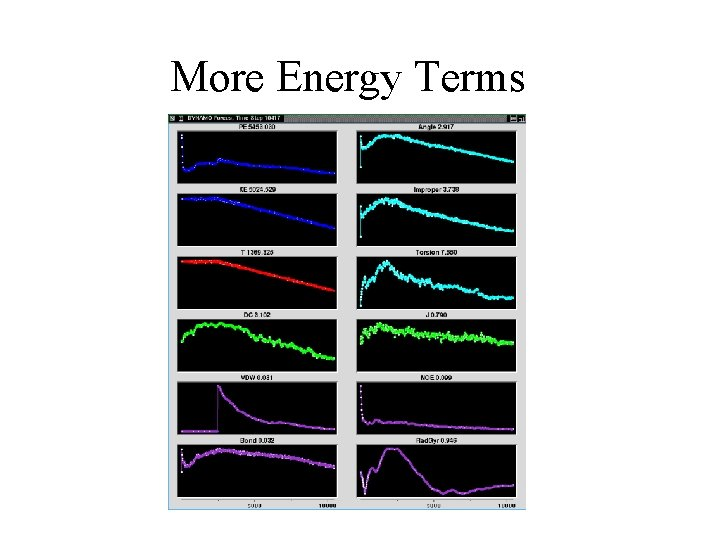More Energy TermsThe Result – a BundleAssessing the Quality of NMR Structures Number of experimental constraints RMSD of structural ensemble (subjective!) Violation of constraints Molecular energies Comparison to structure database: PROCHECK Compactness of Structure, Hydrophobic Burial Back-calculation of experimental parameters Ramachandran Quality